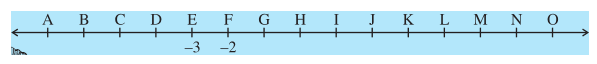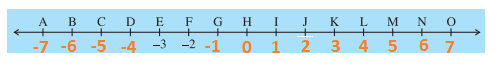## INTRODUCTION

Lesson Progress
0% Complete

INTRODUCTION:

The counting numbers 1, 2, 3, 4…… are called natural numbers. The smallest natural number is 1, but the largest natural number does not exist.

The collection ‘0’ and all natural numbers is called whole numbers. 0 is the smallest whole number. Negatives of counting numbers, i:e. 1, 2, are called negative numbers.

The collection of whole number and negative numbers is called integers.

The smallest or the greatest integer does not exist. −1, −2, are called negative integers and 1, 2, 3, 4, …. are called positive integers.

0 is neither positive nor negative.

On a number line:

a) A number to the right of the given number is greater than the given number.

b) An integer and its opposite are equidistant from 0 but in opposite directions.

WHEN WE

i) Add a positive integer, we move toward right.

ii) Add a negative integer, we move toward left.

iii) Subtract a positive integer, we move towards left.

iv) Subtract a negative integer we move towards right.

Try these: (Page 2)

A number line representing integers is given below.–3 and –2 are marked by E and F respectively.
Which integers are marked by B, D, H, J, M and O?

Solution: Let us complete the given number line such that integers marked by various alphabets are shown. ThereforeThe integer marked by B = 6   The integer marked by D = 4

The integer marked by H =  0   The integer marked by J = 2

The integer marked by M =  5    The integer marked by O =  7

2. Arrange 7, −5, 4, 0 and −4 in ascending order and then mark them on a number line to check your answer.

Solution: Since (i) every positive integer is greater than 0.

(ii) every negative integer is less than 0.

We get –5  < (–4) < 0 < 4 < 7

The required ascending order is  –5,  –4,  0,  4,  7

Since the integer to the right on a number line is greater than that on the left. And the integer on the left is smaller than that on the right.